Author: Tran Duy

Minimum iOS version: 11.0

Graphing calculator provides an UI similar to real graphing calculator 84.
◉ Graphing calculator.
- Calculator supports function, polar and parametric graphs.
- Press MODE and select graph mode, and then press Y= to open graph editor. Press GRAPH to draw these functions.
- Interactive tracing mode, manual tracing mode.
- Show intersection, root and extrema (local maximum, local minimum) of function graph
- Show derivative of function graph
- Show tangent of function and polar graphs
- Best fit line of set of points.
- TABLE generates table of value of selected graph.
- CALC calculates min, max, d/dx, integral of selected graph.

◉ Scientific calculator
- Computer algebra system, symbolic calculation.
- Support all functions of real graphing calculator 83, 84.
- All basic and advanced math functions, complex number, probability, angle, relational operations, logic operations, matrix and vector operations.
- Users can define their calculator function.

◉ Equation solver (Press ALPHA ENTER to open SOLVER menu)
- Solve any equation with auto solver mode, the calculator can give symbolic roots.
- Solve quadratic, cubic, quartic and 5th equation.
- Solve system of equations, up to 5 variables

◉ Statistics
- 1-Var Stats Calculates 1-variable statistics.
- 2-Var Stats Calculates 2-variable statistics.
- LinReg(ax+b) Fits a linear model to data.
- CubicReg Fits a cubic model to data.
- QuartReg Fits a quartic model to data.
- LinReg(a+bx) Fits a linear model to data.
- LnReg Fits a logarithmic model to data.
- ExpReg Fits an exponential model to data.

◉ Inferential Statistics and Distributions
- Z-Test, T-Test, 2-SampZTest, 2-SampTTest, 1-PropZTest, 2-PropZTest, ZInterval, TInterval, 2-SampZInt, 2-SampTInt, 1-PropZInt, 2-PropZInt, Chi2-Test, Chi2-GOF Test, 2-SampFTest, LinRegTTest, LinRegTInt, ANOVA
- All distribution functions, includes normal distribution, student t, Chi-square, F, binomial, ...

statistic, inferential statistics and distributions and a lot of other features.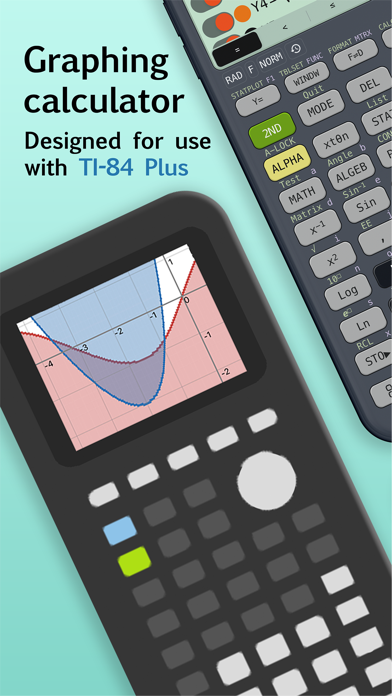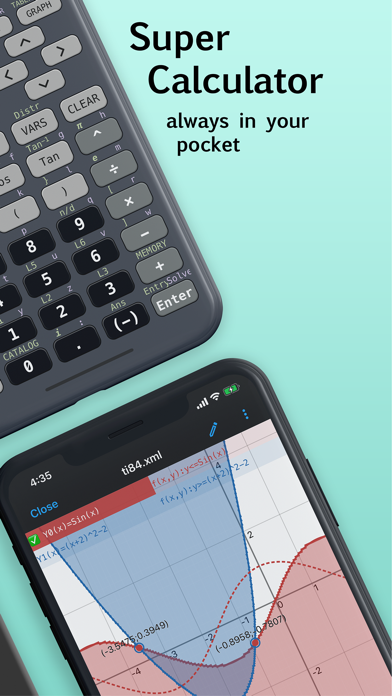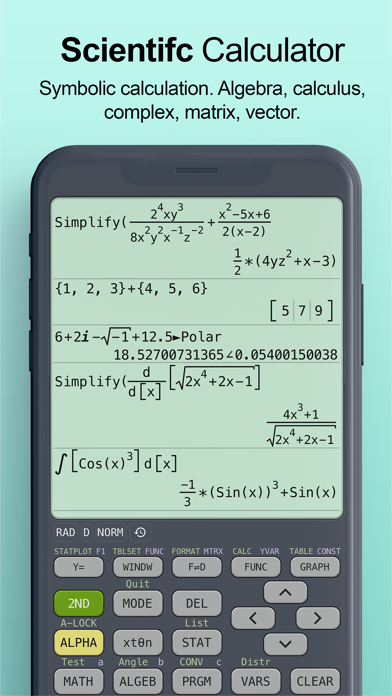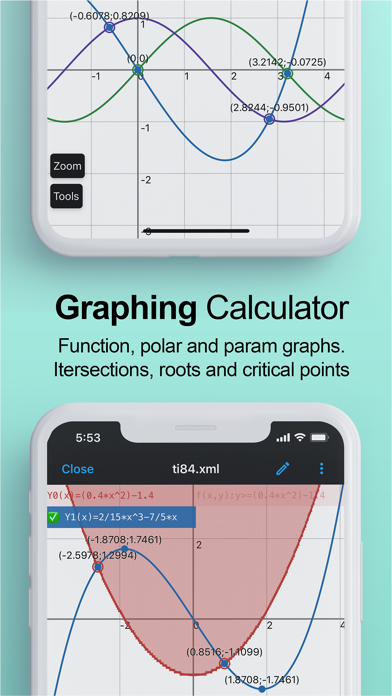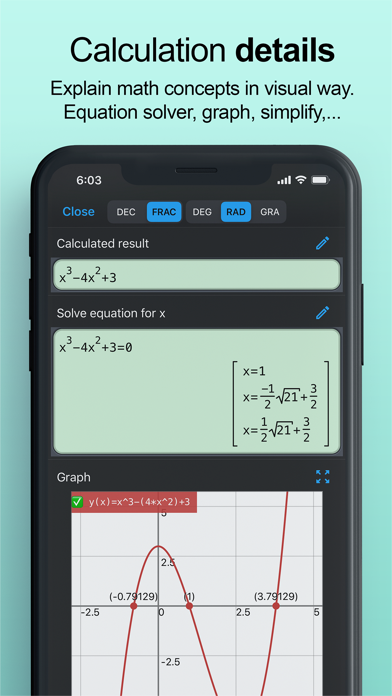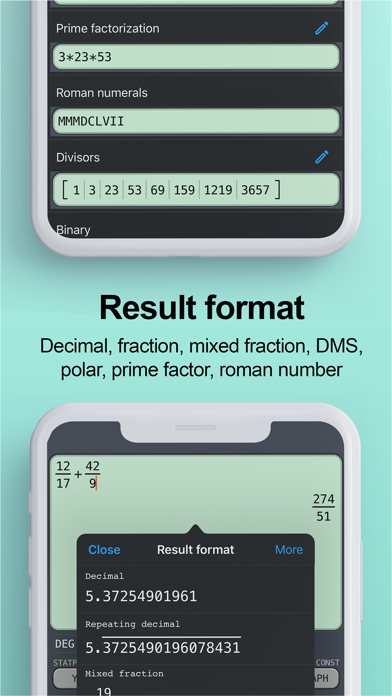bundleId: com.graphing.calc84.plus